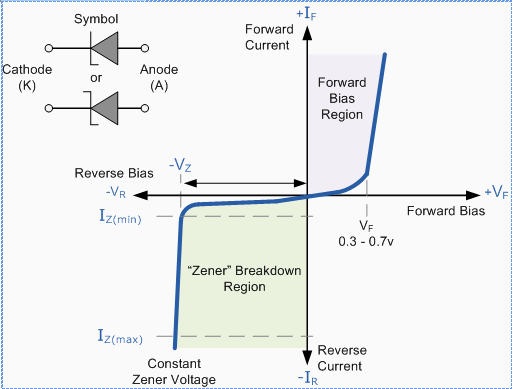Courses

# Zener Diodes Electrical Engineering (EE) Notes | EduRev

## Electrical Engineering (EE) : Zener Diodes Electrical Engineering (EE) Notes | EduRev

The document Zener Diodes Electrical Engineering (EE) Notes | EduRev is a part of the Electrical Engineering (EE) Course Analog Electronics.
All you need of Electrical Engineering (EE) at this link: Electrical Engineering (EE)

ZENER DIODES

The Zener diode is like a general-purpose signal diode consisting of a silicon PN junction. When biased in the forward direction, it behaves just like a normal signal diode passing the rated current, but as soon as a reverse voltage applied across the zener diode exceeds the rated voltage of the device, the diode's breakdown voltage VB is reached (the point at which Avalanche Breakdown occurs)  in the semiconductor depletion layer and a current starts to flow through the diode to limit this increase in voltage.

The current now flowing through the zener diode increases drastically to the maximum circuit value (which is usually limited by a series resistor) and once achieved this reverse saturation current remains fairly constant over a wide range of applied voltage. This breakdown voltage point, VB is called the "zener voltage" for zener diodes and ranges from less than one volt to hundreds of volts.

The point at which the zener voltage triggers the current to flow through the diode can be very accurately controlled (to less than 1% tolerance) in the doping stage of the semiconductor diode construction giving the diode a specific zener breakdown voltage, (Vz) for example, 4.3V or 7.5V. This zener breakdown voltage on the I-V curve is almost a vertical straight line.

Zener Diode I-V CharacteristicsThe Zener Diode is used in its "reverse bias" or reverse breakdown mode, i.e. the anode  of the diode connects to the negative supply. From the I-V characteristics curve above, we can see that the zener diode has a region of almost constant negative voltage in its reverse bias characteristics regardless of the value of the current flowing through the diode and remains nearly constant even with large changes in current as long as the zener diodes current remains between the breakdown current IZ(min) and the maximum current rating IZ(max).

This ability can be used to regulate or stabilise a voltage source against supply or load variations. The fact that the voltage across the diode in the breakdown region is almost constant turns out to be an important application of the zener diode as a voltage regulator. The function of a regulator is to provide a constant output voltage to a load connected in parallel with it in spite of the ripples in the supply voltage or the variation in the load current and the zener diode will continue to regulate the voltage until the diode's current fall below the minimum IZ(min) value in the reverse breakdown region.

Offer running on EduRev: Apply code STAYHOME200 to get INR 200 off on our premium plan EduRev Infinity!

## Analog Electronics

11 videos|56 docs|97 tests

,

,

,

,

,

,

,

,

,

,

,

,

,

,

,

,

,

,

,

,

,

;